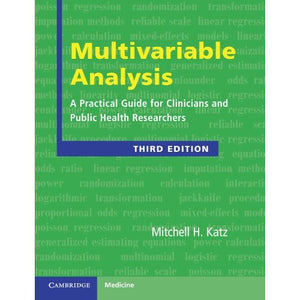># Multivariable Analysis: A Practical Guide for Clinicians and Public Health Researchers

• £26.39
• Save £19

Mitchell H. Katz
Cambridge University Press
Edition: 3, 3/10/2011
EAN 9780521141079, ISBN10: 0521141079

Paperback, 250 pages, 24.6 x 18.9 x 1.3 cm
Language: English

Now in its third edition, this highly successful text has been fully revised and updated with expanded sections on cutting-edge techniques including Poisson regression, negative binomial regression, multinomial logistic regression and proportional odds regression. As before, it focuses on easy-to-follow explanations of complicated multivariable techniques. It is the perfect introduction for all clinical researchers. It describes how to perform and interpret multivariable analysis, using plain language rather than complex derivations and mathematical formulae. It focuses on the nuts and bolts of performing research, and prepares the reader to set up, perform and interpret multivariable models. Numerous tables, graphs and tips help to demystify the process of performing multivariable analysis. The text is illustrated with many up-to-date examples from the medical literature on how to use multivariable analysis in clinical practice and in research.

Preface
1. Introduction
2. Common uses of multivariable models
3. Outcome variables in multivariable analysis
4. Type of independent variables in multivariable analysis
5. Assumptions of multiple linear regression, multiple logistic regression, and proportional hazards analysis
6. Relationship of independent variables to one another
7. Setting up a multivariable analysis
8. Performing the analysis
9. Interpreting the analysis
10. Checking the assumptions of the analysis
11. Propensity scores
12. Correlated observations
13. Validation of models
14. Special topics